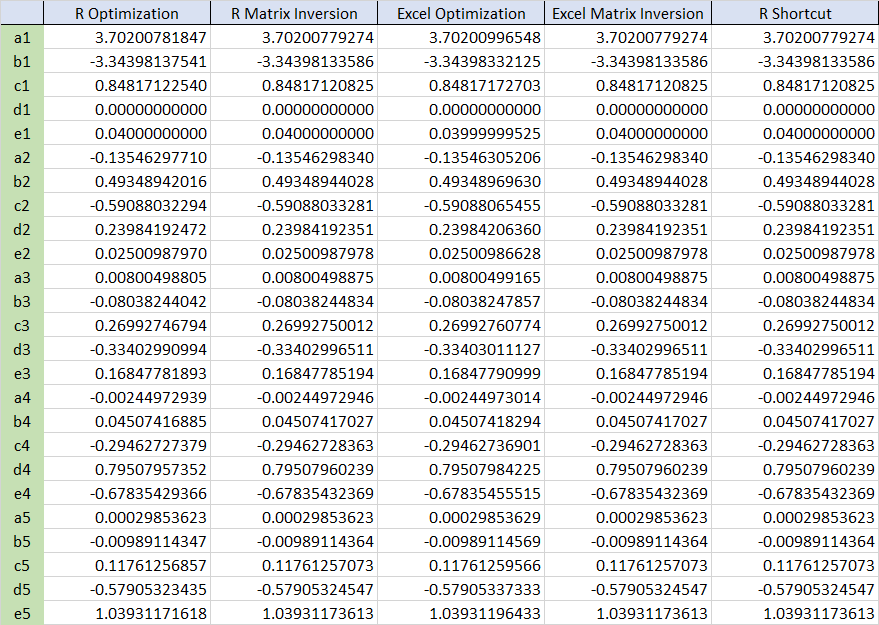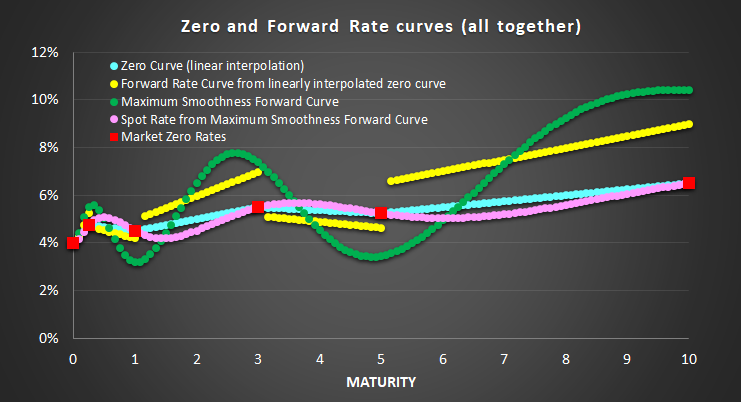# Articles by sang-heon lee

### Regime Switching State Space Model with R code

February 27, 2022 |

This post explains a Markov regime switching state space model. The bottom line is two-fold: 1) expanding states by each regime transitions and 2) collapsing each updated estimates for the next state prediction. The step 2) is necessary to fix t...### Kim (1994) Smoother Algorithm in Regime Switching Model using R code

February 19, 2022 |

This post explains smoothing algorithm of a regime switching model, which is known as Kim (1994) smoother. It is known that smoothing algorithm is more difficult to understand than filtering algorithm. For this perspective, I give detailed deriv...### Hamilton Regime Switching Model using R code

February 17, 2022 |

This post estimates parameters of a regime switching model directly by using R code. The same model was already implemented by using MSwM R package in the previous post. Through this hand-on example I hope we can learn the process of Hamilton fi...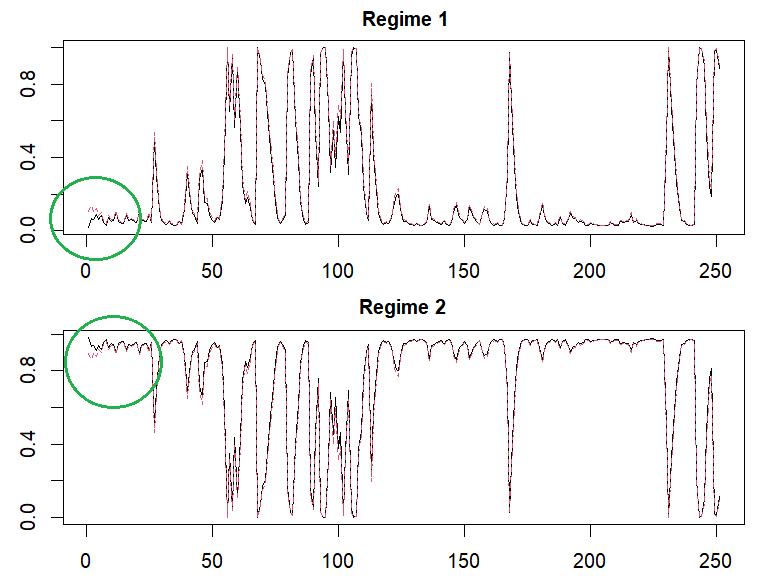### Understanding Hamilton Regime Switching Model using R package

February 13, 2022 |

This post explains how to model a regime switching (Markov switching) model which is based on Hamilton (1989). the estimation is done by 1) predicting states by each regimes, 2) constructing a likelihood from data and state predictions, and 3) u...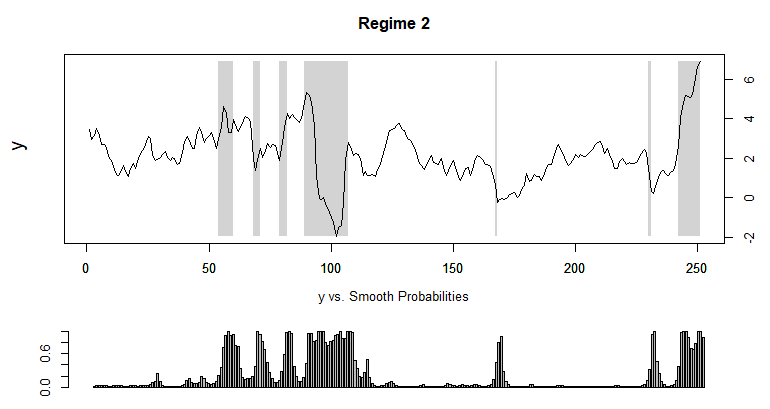### Carter-Kohn algorithm for State Space Models in R : Univariate Case

February 6, 2022 |

This post implements a R code for Carter-Kohn algorithm in a simple univariate state space model. For clear understanding of this backward sampling algorithm, only Gibbs sampling for state estimates is considered with other parameters being fixed or known. Gibbs Sampling for State Space Models in R In the previous ...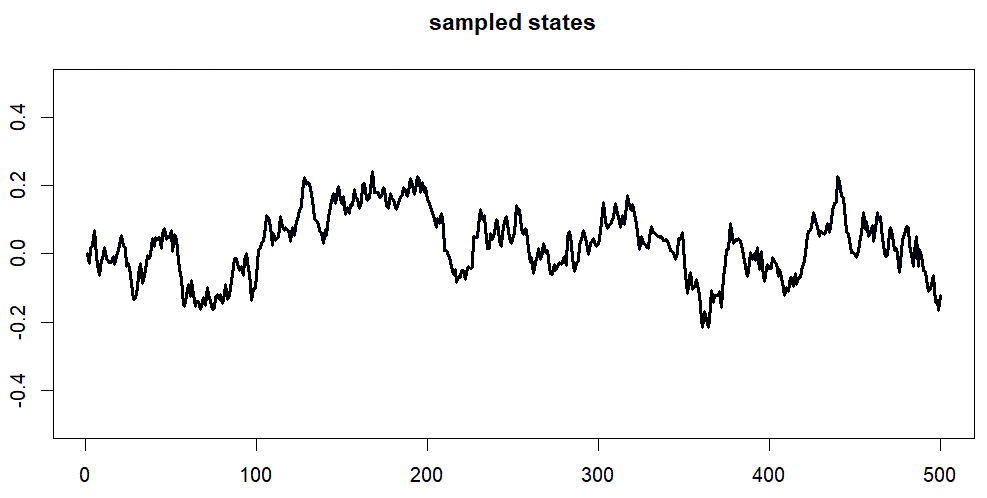### Uncovered Interest Rate Parity and F-test on Regression Parameters using R

January 2, 2022 |

This post explains how to perform the F-test of joint parameter restrictions on a linear regression model. As an example, we use the data in Chen and Tsang (2013), who introduce so called relative Nelson-Siegel factor model to predict exchange r...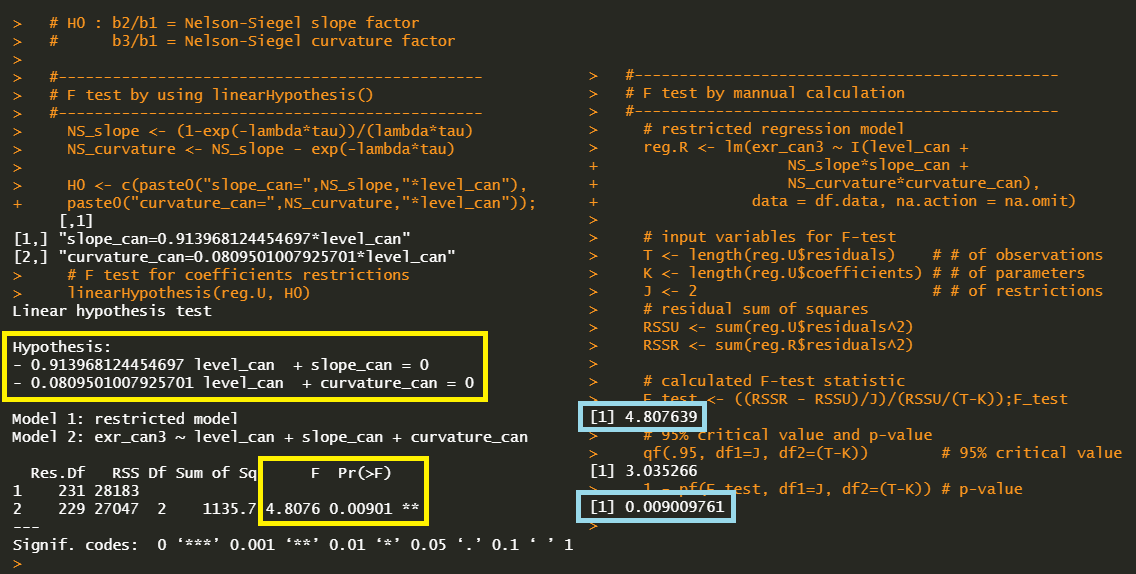### Some Interesting Issues in VECM using R

December 28, 2021 |

This post deals with some interesting issues regarding the VECM model. Among them are the VAR representation of VECM, the weak exogeniety restrictions and user-defined cointegrating vectors and so on. With the help of useful R packages, these is...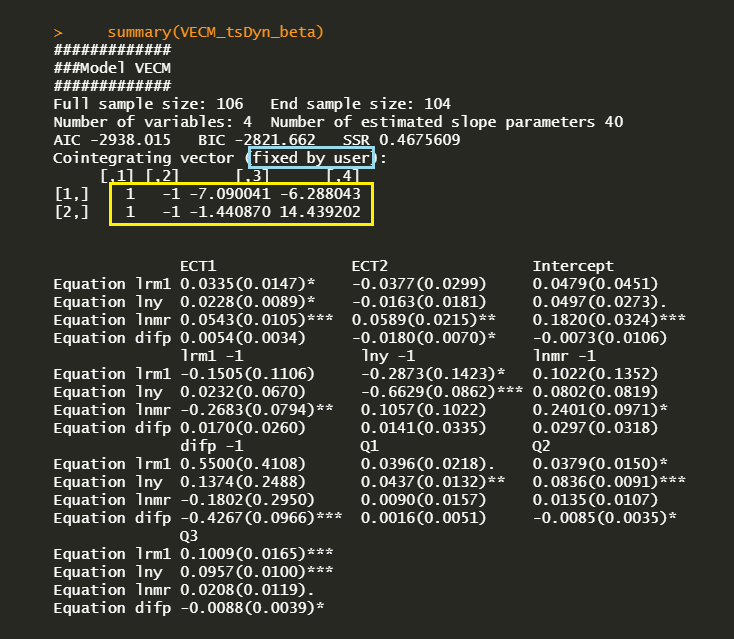### Vector Error Correction Model (VECM) using R

December 24, 2021 |

This post explains how to estimate and forecast a Vector Error Correction Model (VECM) model using R. The VECM model consists of VAR model (short-term dynamics) and cointegration (long-term comovement). We use the Johansen cointegration test. The co...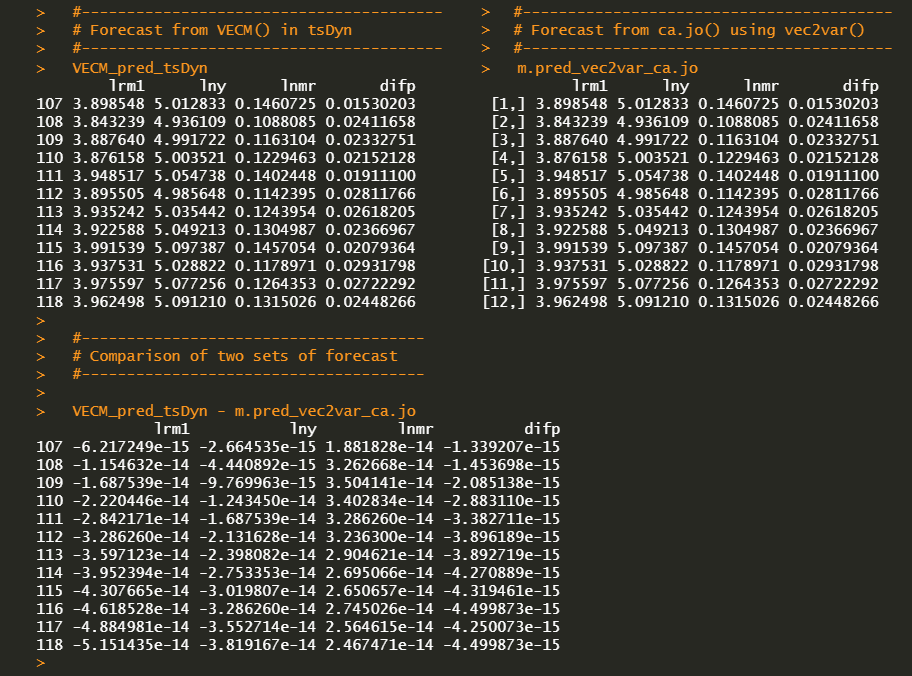### Pastor and Stambaugh (2003) Liquidity Measure – Individual Stock

December 10, 2021 |

This post shows how to calculate the liquidity measure (gamma) of Pastor and Stambaugh (2003) using R code. For exposition purposes we use only two individual series : small firm index and ESG firm index. As expected, a PS liquidity measure for smal...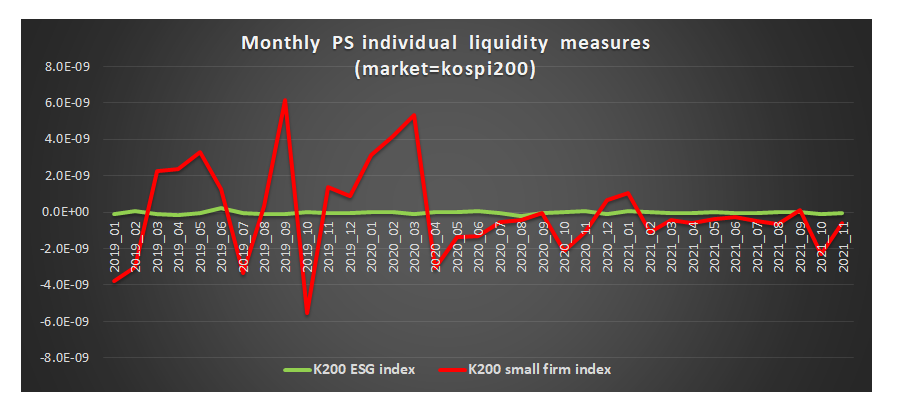### Easy Interpretations of ADF Test in R

December 5, 2021 |

This post shows how to interpret the results of the augmented Dickey-Fuller (ADF) test easily with the help of Hank Roark's R function. His R function provides kind descriptions of the results of a unit root ADF test. I explains why this description...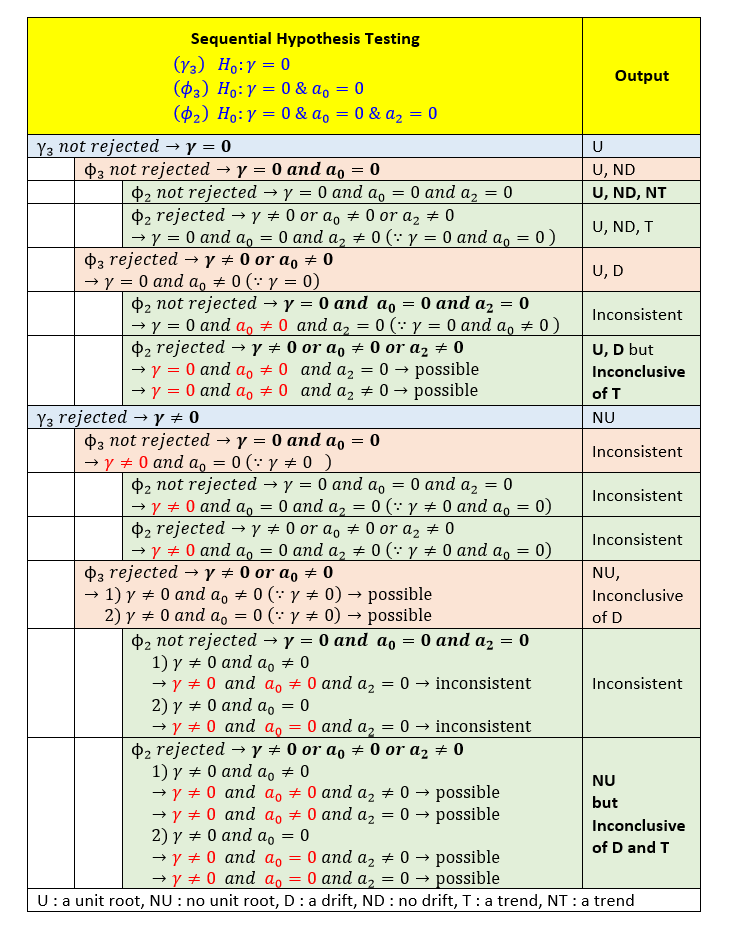### Augmented Dickey-Fuller (ADF) Test in R

December 4, 2021 |

This post explains how to use the augmented Dickey-Fuller (ADF) test in R. The ADF Test is a common statistical test to determine whether a given time series is stationary or not. We explain the interpretation of ADF test results from R package by m...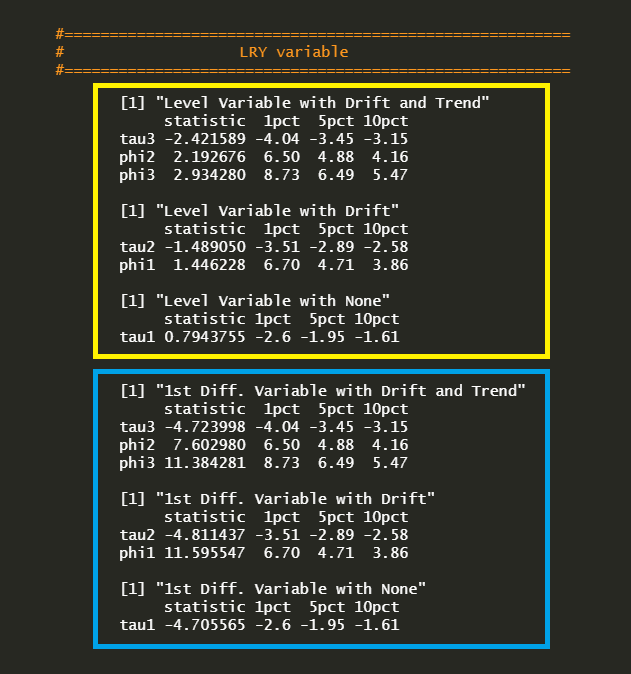### Vector Autoregressive Model (VAR) using R

November 28, 2021 |

This post gives a brief introduction to the estimation and forecasting of a Vector Autoregressive Model (VAR) model using R . We use vars and tsDyn R package and compare these two estimated coefficients. We also consider VAR in level and VAR in diff...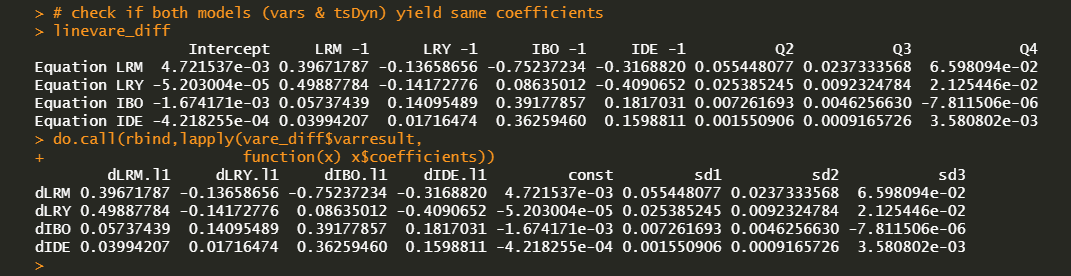### Bond Forward Pricing using R code

November 6, 2021 |

This post presents a R code for pricing a bond forward. It is well known that insurance companies use it as the instrument of a duration management with lower cost. Here, a pricing formula and its implementation are provided with delta sensitivity u...### lambda.min, lambda.1se and Cross Validation in Lasso : Continuous Response

November 1, 2021 |

This post presents a R code for a k-fold cross validation of Lasso in the case of a gaussian regression (continuous Y). This work easily can be done by using a mean squared error. Cross Validation in Lasso : Gaussian Regression We have implem...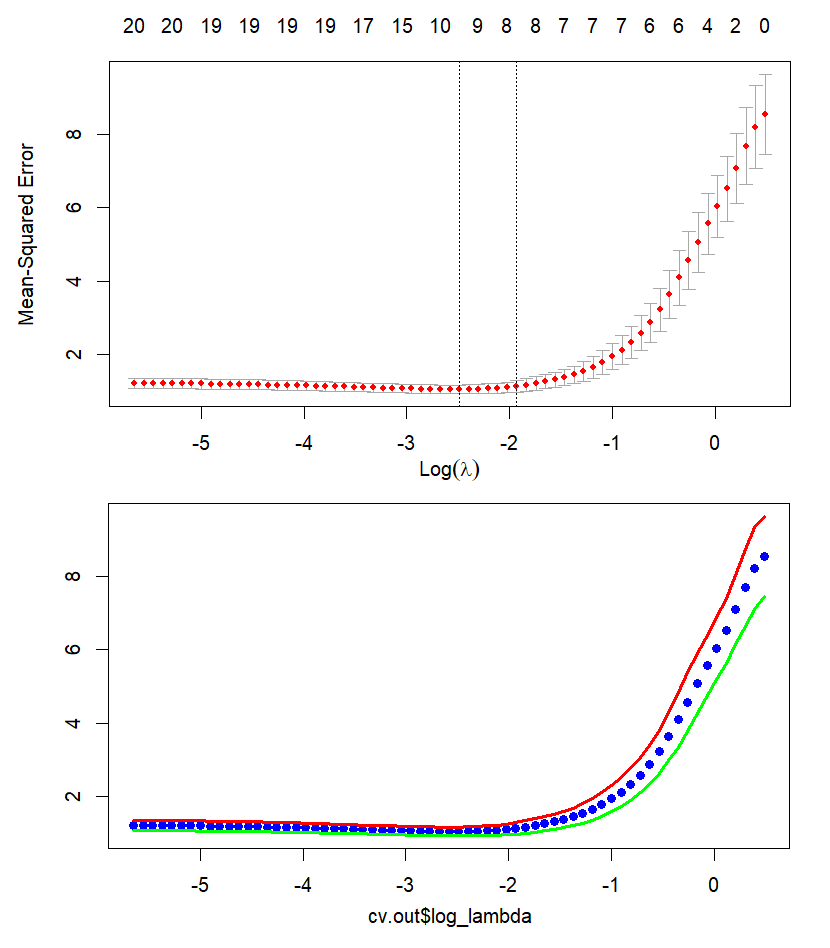### lambda.min, lambda.1se and Cross Validation in Lasso : Binomial Response

October 30, 2021 |

This post explains more details regarding cross validation of Lasso in the case of a binomial response. We implement a R code for a lasso model's cross validation. In addition, We calculate lambda.min and lambda.1se manually. Finally we compare thes...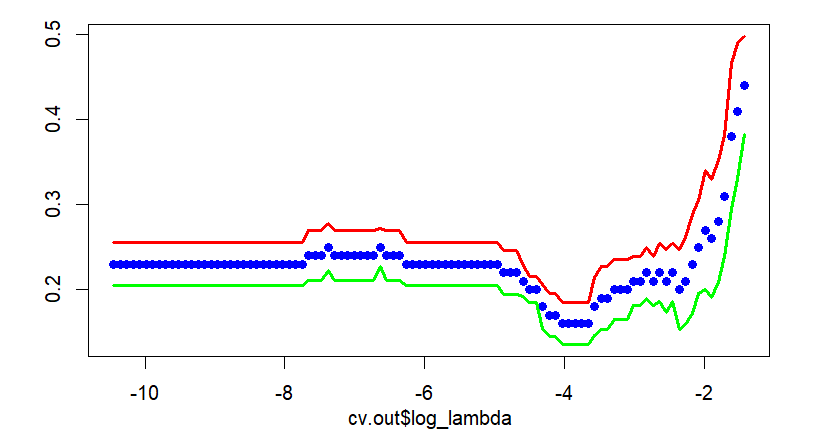### Exclusive Lasso and Group Lasso using R code

October 28, 2021 |

This post shows how to use the R packages for estimating an exclusive lasso and a group lasso. These lasso variants have a given grouping order in common but differ in how this grouping constraint is functioning when a variable selection is performe...### R : httr in R and MSXML2.ServerXMLHTTP in Excel VBA

October 27, 2021 |

This post shows a R counterpart of Excel VBA's MSXML2.ServerXMLHTTP related commands with which server APIs are called easily. In case of R, it is done by using httr R package. As an illustration, SQL query for retrieving swap date schedule is execu...### Robustness Check for Maximum Smoothness Forward Rates using R

October 17, 2021 |

This post makes a user-defined R function for Adams and Deventer (1994, revised 2010) maximum smoothness forward curve for the purpose of reusing and performs robustness checks for various initial term structures. We find that every time an initial ...### Maximum Smoothness Forward Rates by Inverse Matrix using R

October 16, 2021 |

This post solves Adams and Deventer (1994) maximum smoothness forward rate curve as the matrix inversion of equality constrained quadratic programming problems. We implement R code for this model and elaborate on how to get monthly forward and spot ...